NCERT Exemplar MCQ

Chapter 7 Class 12 Integrals
Serial order wise

## (D) 4 log⁡2

This question is similar to Question 20 - CBSE Class 12 - Sample Paper for 2019 Board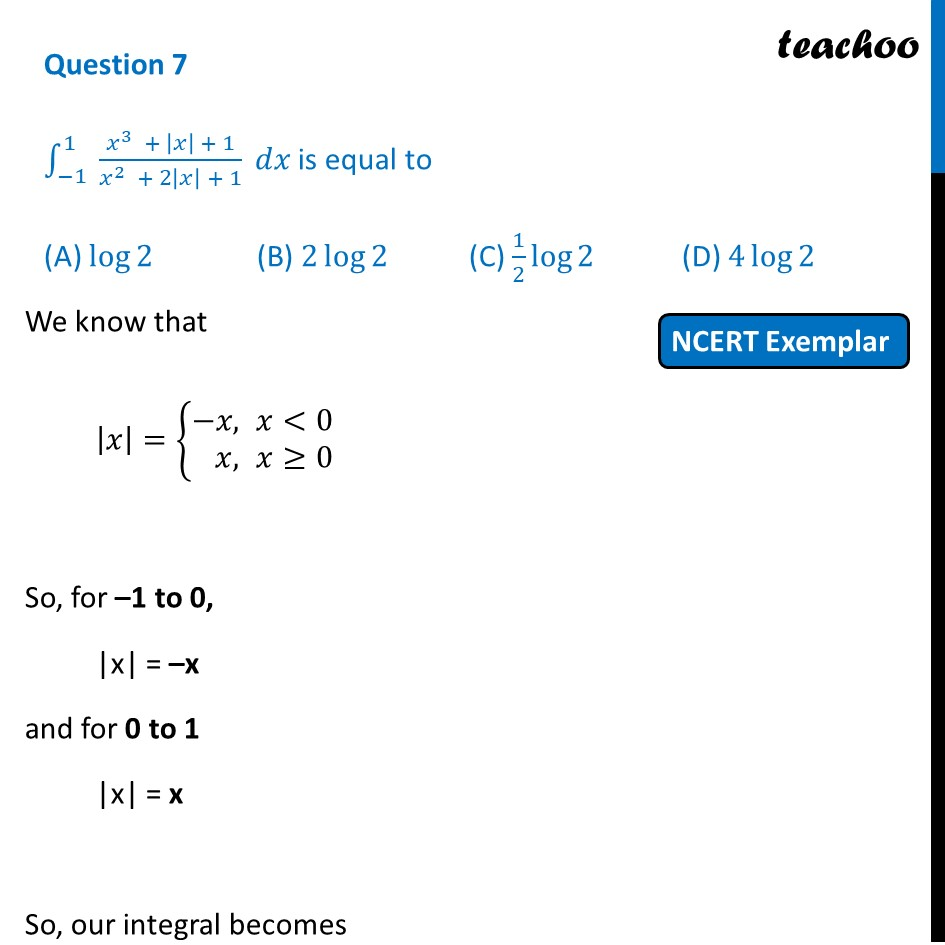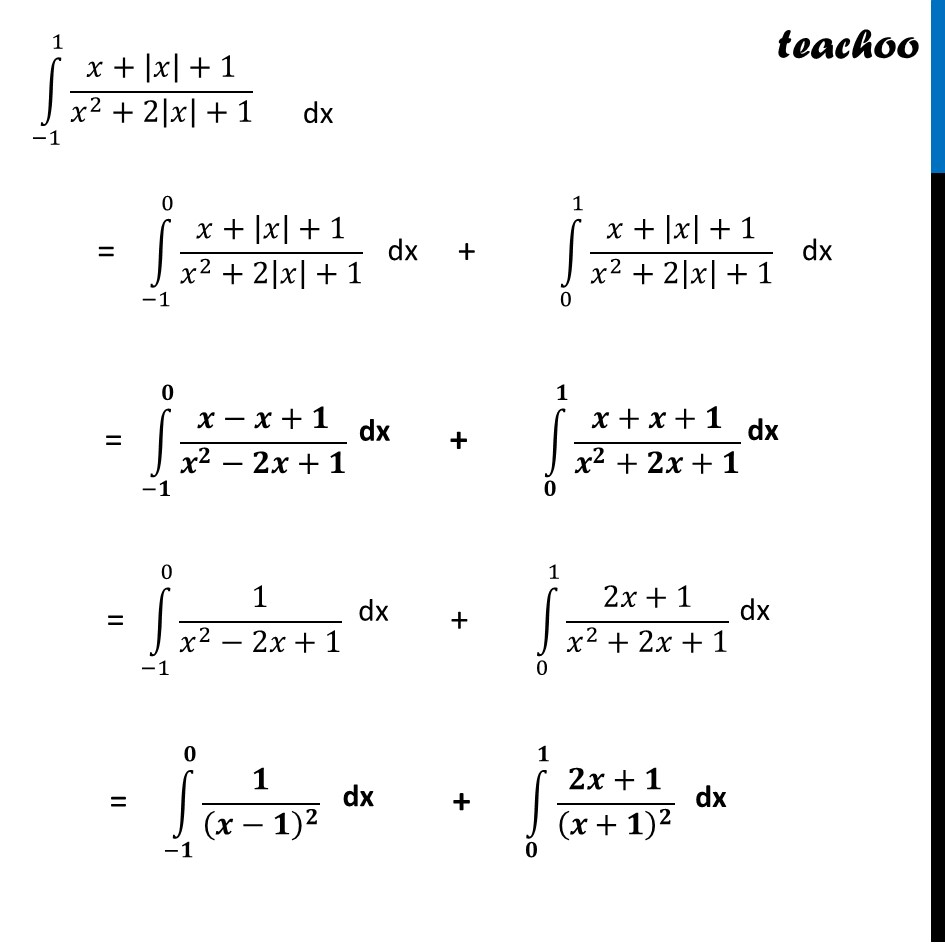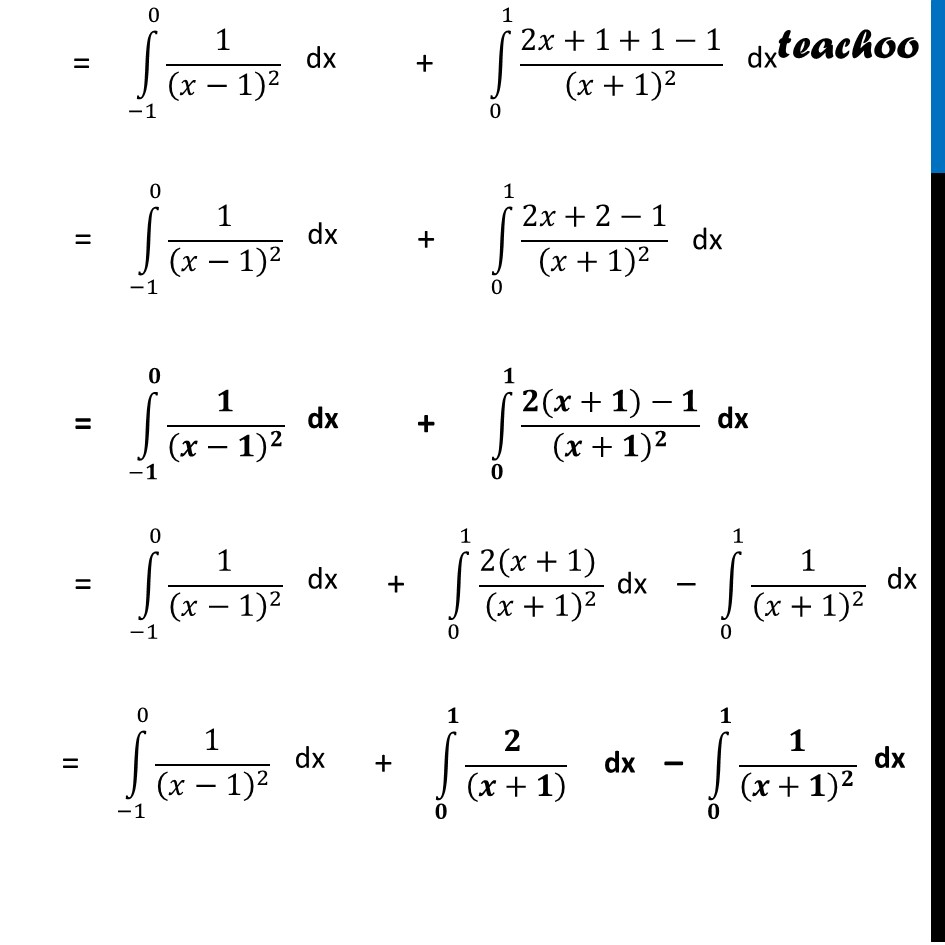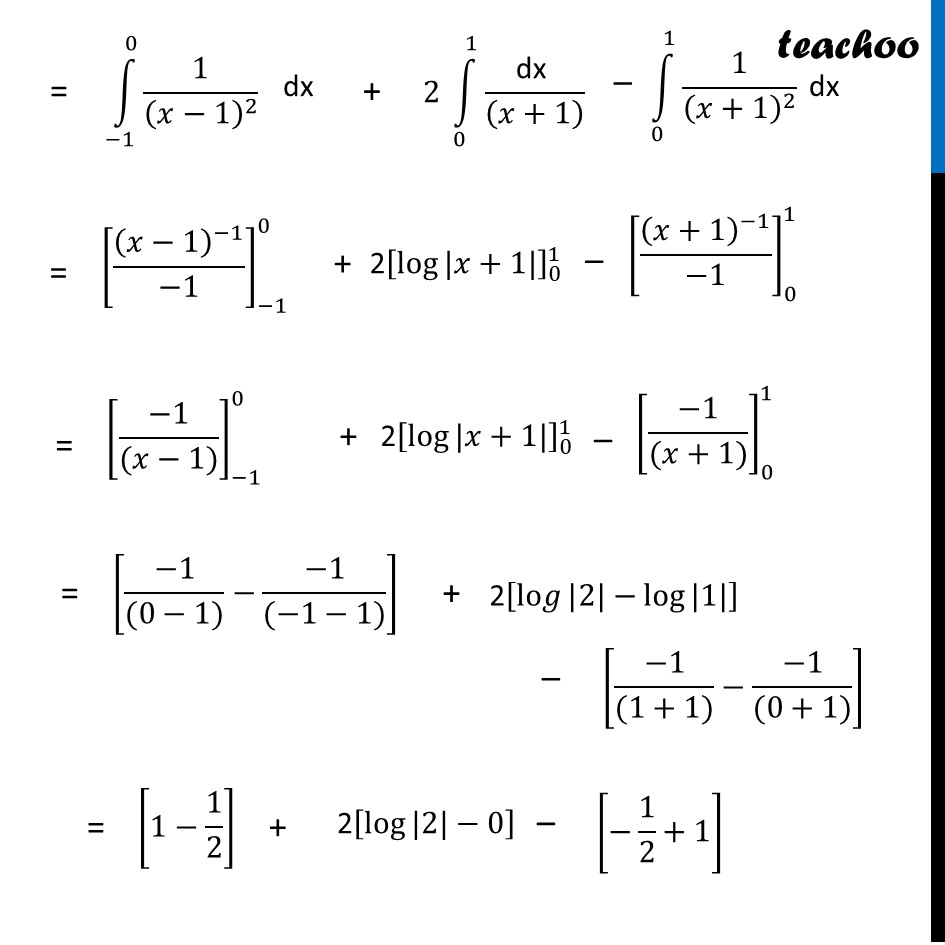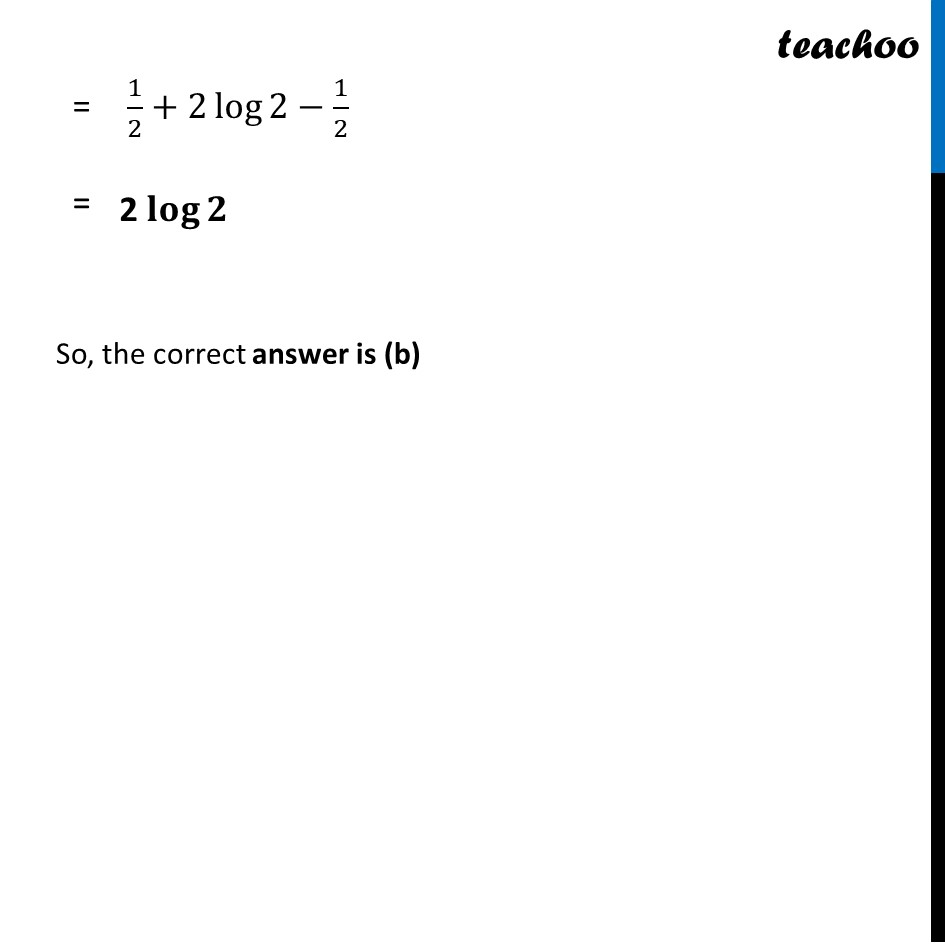Learn in your speed, with individual attention - Teachoo Maths 1-on-1 Class

### Transcript

Question 7 ∫1_(−1)^1▒〖 (𝑥^3 + |𝑥| + 1)/(𝑥^2 + 2|𝑥| + 1)〗 𝑑𝑥 is equal to (A) log⁡2 (B) 2 log⁡2 (C) 1/2 log⁡2 (D) 4 log⁡2 We know that |𝑥|={█(−&𝑥, 𝑥<0@&𝑥, 𝑥≥0)┤ So, for –1 to 0, |x| = –x and for 0 to 1 |x| = x So, our integral becomes ∫1_(−1)^1▒(𝑥+|𝑥|+1)/(𝑥^2+2|𝑥|+1) ∫1_(−1)^0▒(𝑥+|𝑥|+1)/(𝑥^2+2|𝑥|+1) ∫1_0^1▒(𝑥+|𝑥|+1)/(𝑥^2+2|𝑥|+1) ∫1_(−𝟏)^𝟎▒(𝒙−𝒙+𝟏)/(𝒙^𝟐−𝟐𝒙+𝟏) ∫1_𝟎^𝟏▒(𝒙+𝒙+𝟏)/(𝒙^𝟐+𝟐𝒙+𝟏) ∫1_(−1)^0▒1/(𝑥^2−2𝑥+1) ∫1_0^1▒(2𝑥+1)/(𝑥^2+2𝑥+1) ∫1_(−𝟏)^𝟎▒𝟏/(𝒙−𝟏)^𝟐 ∫1_𝟎^𝟏▒(𝟐𝒙+𝟏)/(𝒙+𝟏)^𝟐 ∫1_(−1)^0▒1/(𝑥−1)^2 ∫1_0^1▒(2𝑥+1+1−1)/(𝑥+1)^2 ∫1_0^1▒(2𝑥+1+1−1)/(𝑥+1)^2 ∫1_(−1)^0▒1/(𝑥−1)^2 ∫1_0^1▒(2𝑥+2−1)/(𝑥+1)^2 ∫1_(−𝟏)^𝟎▒𝟏/(𝒙−𝟏)^𝟐 ∫1_𝟎^𝟏▒(𝟐(𝒙+𝟏)−𝟏)/(𝒙+𝟏)^𝟐 ∫1_(−1)^0▒1/(𝑥−1)^2 ∫1_0^1▒(2(𝑥+1) )/(𝑥+1)^2 ∫1_0^1▒1/(𝑥+1)^2 ∫1_(−1)^0▒1/(𝑥−1)^2 ∫1_𝟎^𝟏▒(𝟐 )/((𝒙+𝟏) ) ∫1_𝟎^𝟏▒𝟏/(𝒙+𝟏)^𝟐 ∫1_(−1)^0▒1/(𝑥−1)^2 2 ∫1_0^1▒"dx " /((𝑥+1) ) ∫1_0^1▒1/(𝑥+1)^2 [(𝑥−1)^(−1)/(−1)]_(−1)^0 2[log⁡〖|𝑥+1|〗 ]_0^1 [(𝑥+1)^(−1)/(−1)]_0^1 1/2+2 log⁡2−1/2 2 𝐥𝐨𝐠⁡𝟐 So, the correct answer is (b)